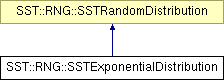# SST::RNG::SSTExponentialDistribution Class Reference

Creates an exponential distribution for use within SST. More...

`#include "sst/core/rng/expon.h"`

Inheritance diagram for SST::RNG::SSTExponentialDistribution:## Public Member Functions

SSTExponentialDistribution (const double mn)
Creates an exponential distribution with a specific lambda.
SSTExponentialDistribution (const double mn, SSTRandom *baseDist)
Creates an exponential distribution with a specific lambda and a base random number generator.
~SSTExponentialDistribution ()
Destroys the exponential distribution.
double getNextDouble ()
Gets the next (random) double value in the distribution.
double getLambda ()
Gets the lambda with which the distribution was created.

## Protected Attributes

double lambda
Sets the lambda of the exponential distribution.
SSTRandombaseDistrib
Sets the base random number generator for the distribution.
bool deleteDistrib
Controls whether the base distribution should be deleted when this class is destructed.

## Detailed Description

Creates an exponential distribution for use within SST.

This distribution is the same across platforms and compilers.

## Constructor & Destructor Documentation

 SST::RNG::SSTExponentialDistribution::SSTExponentialDistribution ( const double mn ) ` [inline]`

Creates an exponential distribution with a specific lambda.

Parameters:
 mn The lambda of the exponential distribution

References baseDistrib, deleteDistrib, and lambda.

 SST::RNG::SSTExponentialDistribution::SSTExponentialDistribution ( const double mn, SSTRandom * baseDist ) ` [inline]`

Creates an exponential distribution with a specific lambda and a base random number generator.

Parameters:
 mn The lambda of the exponential distribution baseDist The base random number generator to take the distribution from.

References baseDistrib, deleteDistrib, and lambda.

## Member Function Documentation

 double SST::RNG::SSTExponentialDistribution::getLambda ( ) ` [inline]`

Gets the lambda with which the distribution was created.

Returns:
The lambda which the user created the distribution with

References lambda.

 double SST::RNG::SSTExponentialDistribution::getNextDouble ( ) ` [inline, virtual]`

Gets the next (random) double value in the distribution.

Returns:
The next random double from the distribution

Implements SST::RNG::SSTRandomDistribution.

References baseDistrib, lambda, and SST::RNG::SSTRandom::nextUniform().

The documentation for this class was generated from the following file:

Generated on 14 Sep 2015 for SST by1.6.1